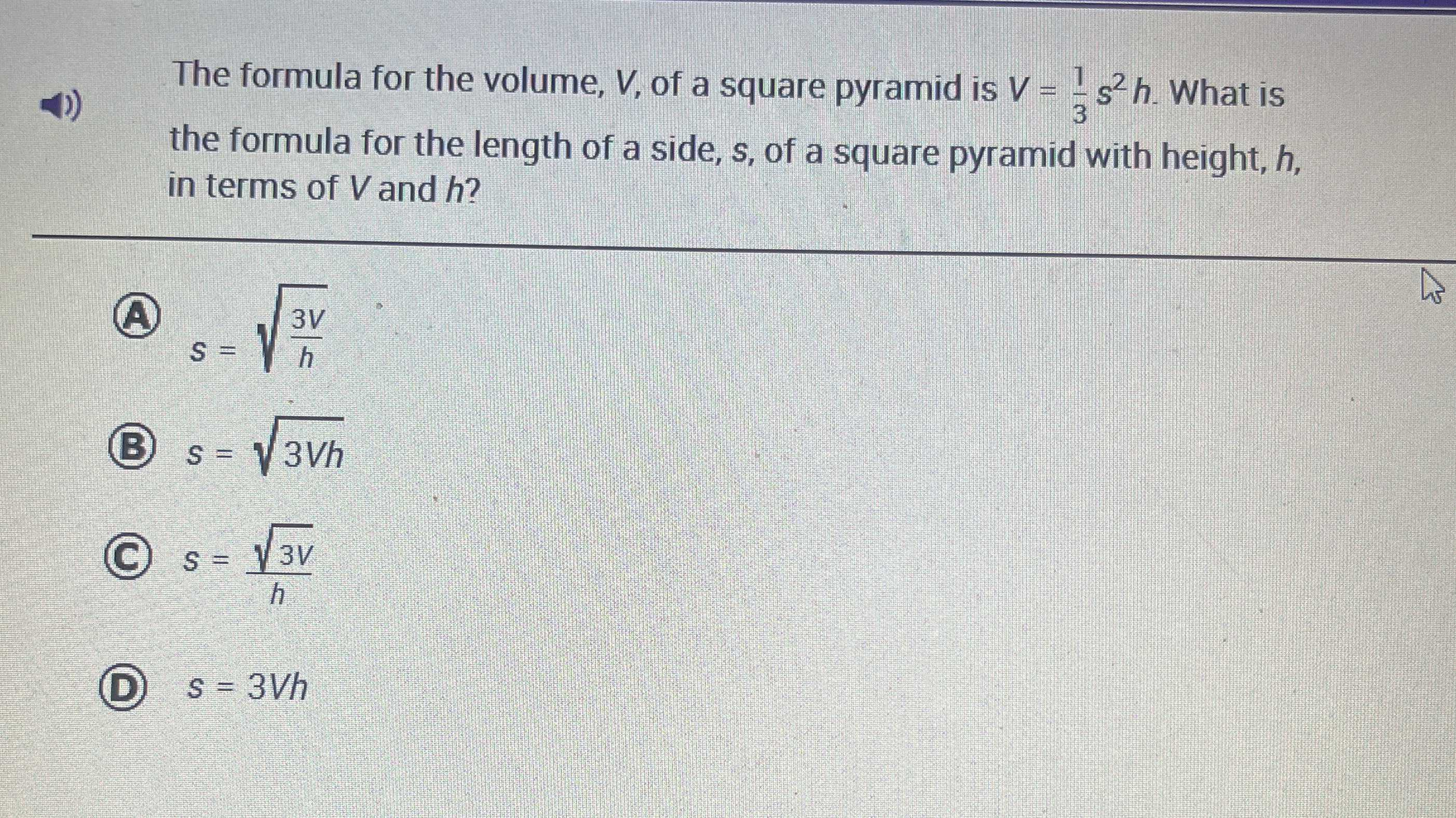### Still have math questions?

Algebra
QuestionThe formula for the volume, $$V$$ , of a square pyramid is $$V = \frac { 1 } { 3 } s ^ { 2 } h$$ . What is the formula for the length of a side, $$s$$ , of a square pyramid with height, $$h$$ , in terms of $$V$$ and $$h$$ ?

(A) $$s = \sqrt { \frac { 3 V } { h } }$$

(B) $$s = \sqrt { 3 V h }$$

(C) $$s = \frac { \sqrt { 3 V } } { h }$$

(D) $$s = 3 V h$$